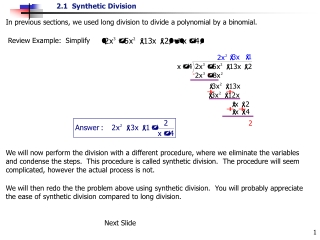DownloadDownload PresentationIn previous sections, we used long division to divide a polynomial by a binomial.

# In previous sections, we used long division to divide a polynomial by a binomial.

Download Presentation## In previous sections, we used long division to divide a polynomial by a binomial.

- - - - - - - - - - - - - - - - - - - - - - - - - - - E N D - - - - - - - - - - - - - - - - - - - - - - - - - - -
##### Presentation Transcript

1. In previous sections, we used long division to divide a polynomial by a binomial. Review Example: Simplify We will then redo the the problem above using synthetic division. You will probably appreciate the ease of synthetic division compared to long division. Next Slide We will now perform the division with a different procedure, where we eliminate the variables and condense the steps. This procedure is called synthetic division. The procedure will seem complicated, however the actual process is not.

2. Procedure: To divide a polynomial P(x) by a binomial x  c Step 11. The row below the horizontal bar is the quotient (answer). The first number is the leading coefficient with the degree (variables exponent) one less than the degree of the variable from original dividend. The last number is the remainder. The second to last number is the constant. Next Slide Step 1. Arrange the coefficients of P(x) in order of descending power of x inside the division bar. Write 0 for each missing power. Step 2. Write ‘c’ directly in front of the division bar. Step 3. Draw in a horizontal line below the coefficients of P(x) with enough room to write numbers between them. Step 4. Bring down the first coefficient of P(x) below the horizontal line. Step 5. Multiply ‘c’ with the number brought down from P(x). Write this result directly underneath the next coefficient of P(x). Step 6. Add vertically these two numbers. Step 7. Continue this process. Multiply ‘c’ with the 2nd number on this very bottom row. Write result under the 3rd number of P(x). Step 8. Add vertically those two numbers. Step 9. Continue this process until the last column has been added. Step 10. The last vertical sum is the remainder of the quotient.

3. Example 1. Use Synthetic Division to determine the quotient and remainder. remainder Your Turn Problem #1 Use Synthetic Division to determine the quotient and remainder. 1. Rewrite coefficients inside division box. • Write ‘c’ in front of division box. (Always use the opposite sign of the constant.) 3. Horizontal bar. 2 2 4. Bring down 1st coefficient. 5. Multiply that number by ‘c’, place under 2nd number. 6. Add vertically. 7. Multiply ‘c’ by this number. Write it under the 3rd column. 8. Add vertically. • Continue the process until the last column has been added. 10. The last number is the remainder. • Now write the quotient in proper form. The exponent of the first variable is 1 less than the degree of the dividend.

4. Example 2. Use Synthetic Division to determine the quotient and remainder. 3 remainder Your Turn Problem #2 Use Synthetic Division to determine the quotient and remainder. 1. Rewrite coefficients inside division box. 2. Write ‘c’ in front of division box. (Always use the opposite sign of the constant.) 2 7 3. Bring down 1st coefficient. 4. Multiply that number by ‘c’, place under 2nd number. 5. Add vertically. 6. Continue the process until the last column has been added. 7. The last number is the remainder. 8. Now write the quotient in proper form. The exponent of the first variable is 1 less than the degree of the dividend.

5. Final Notes: Write the polynomial in descending order if it is not already. If a terms is missing, i.e., if there is no x2 term, place a zero for the coefficient inside the division box in the correct spot. ‘c’ is obtained from the divisor: x  c. If the divisor is x  7, then c = 7. If the divisor is x+ 4, then c =  4 because x + 4 = x  (4). Example 3. Use Synthetic Division to determine the quotient and remainder. 2 remainder Your Turn Problem #3 Use Synthetic Division to determine the quotient and remainder. • Rewrite P(x) in descending order, then write coefficients inside and c outside the division box. 2. Perform the algorithm (procedure). 0

6. Example 4. Use Synthetic Division to determine the quotient and remainder. Solution: 1 Your Turn Problem #4 Use Synthetic Division to determine the quotient and remainder. 27 0 The End. B.R. 2-21-07